Search

Finding the Inverse of a Function (page 6 of 7)

Sections: Definition / Inverting a graph, Is the inverse a function?, Finding inverses, Proving inverses

• Find the inverse of f(x) = sqrt[ 4  x2 ], 2 < x < 0

 Without the domain restriction, the graph looks like this:    This clearly fails the Horizontal Line Test, so the inverse, without the domain restriction, would not be a function.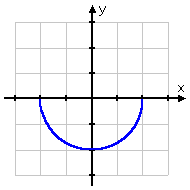Copyright © Elizabeth Stapel 2000-2011 All Rights Reserved However, with the domain restriction, I get this: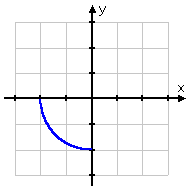The restricted function passes the Horizontal Line Test, so the inverse will now be a function. Since the domain of the original function is 2 < x < 0 and the range is 2 < y < 0, then the domain of the inverse will be 2 < x < 0 and the range will be 2 < y < 0. Yes, the domains and the ranges are identical. Here's the algebra:

 The original function: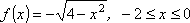I rename "f(x)" as "y":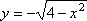Then I solve for "x =":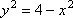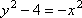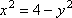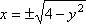Since I already figured out the domain and range, I know that I have to pick the NEGATIVE square root here: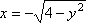Now I switch x and y: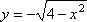And rename "y" as "f-inverse".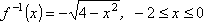So the inverse is the exact same function I started with!

 If you think about it, this makes perfect sense. The original function was one quarter of the circle centered at the origin and having radius r = 2. Considering where the reflecting line y = x goes, and the fact that the points on either side of the reflecting line are neatly symmetric, then the inverse couldn't be anything other than what we got.If you use the upper-right quarter of the circle you'll get the same result; the inverse will be identical to the function. However, if you take either of the other two quarters, you'll get the remaining quarter as the inverse: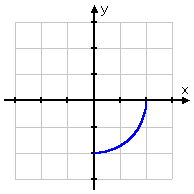...and: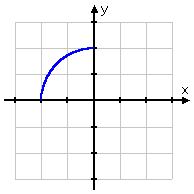<< Previous  Top  |  1 | 2 | 3 | 4 | 5 | 6 | 7  |  Return to Index  Next >>

 Cite this article as: Stapel, Elizabeth. "Finding the Inverse of a Function." Purplemath. Available from     https://www.purplemath.com/modules/invrsfcn6.htm. Accessed [Date] [Month] 2016

Study Skills Survey

Tutoring from Purplemath
Find a local math tutor

 Copyright © 2021  Elizabeth Stapel   |   About   |   Terms of Use   |   Linking   |   Site Licensing Contact Us